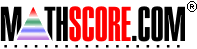Math Practice Online > free > lessons > Florida > 6th grade > Triangle Angles 2

## Triangle Angles 2

This topic covers the idea of finding missing angles using properties of supplementary angles

 Sample Problems for Triangle Angles 2 Lesson for Triangle Angles 2

### This topic aligns to the following state standards

Grade 8: Geo 1. Determines and justifies the measures of various types of angles based upon geometric relationships in two- and three-dimensional shapes.
Grade 7: Geo 2. Creates and solves angle measurement problems for triangles.
Grade 9: Geo 1. represents and applies geometric properties and relationships to solve real-world and mathematical problems including ratio, proportion, and properties of right triangle trigonometry

Copyright Accurate Learning Systems Corporation 2008.